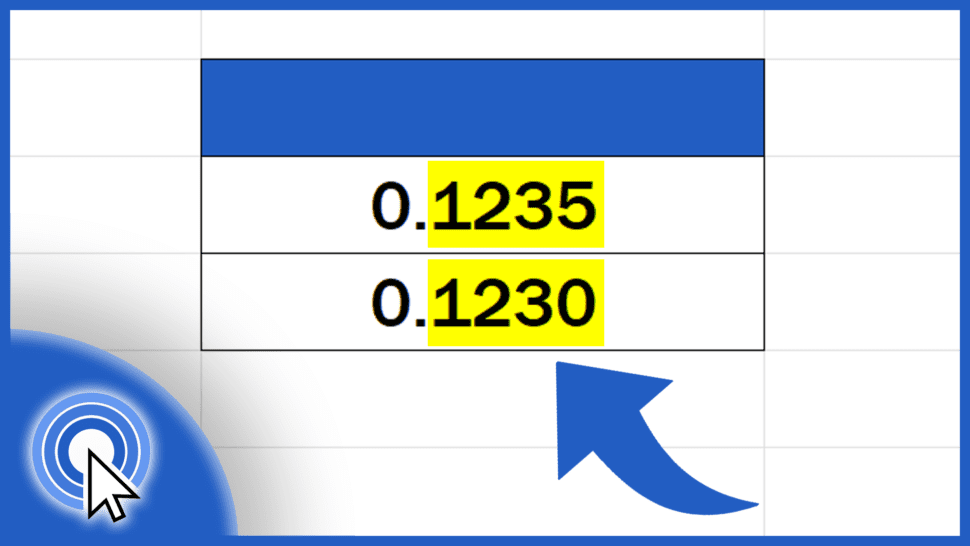# How to Change the Number of Decimal Places in Excel (Two Most Common Ways)Today we’re going to talk about two most common ways how to change the number of decimal places in Excel.

Would you rather watch this tutorial? Click the play button below!

Contents

## The First Way How to Change the Number of Decimal Places in Excel (Using Options ‘Increase Decimal’ and ‘Decrease Decimal’)

The first (and the fastest) way how to change the number of decimal places in Excel is to use the buttons in the Home tab, specifically the options ‘Increase Decimal’ and ‘Decrease Decimal’.

To do that, we need to select the cells where the number of decimal places should be changed. To increase the number of decimal places, click on ‘Increase Decimal’.

Here, Excel unifies the number of decimal places for both cells. If we increase the number of decimal places to more than the numbers actually contain, Excel adds zeros to make up the count.

Now, let’s do it the other way round and let’s decrease the number of decimal places. For that, we need to use the button ‘Decrease Decimal’. Here we need to keep in mind that if we decrease the number of the decimal places, Excel will round the figures.

Let’s say B3 contains a figure that consists of the number 6 located on the sixth decimal place. Based on this, if we decrease the number of decimal places to five, the number 5 located on the fifth decimal place will round to 6.

It’s also important to remember that after decreasing the decimal places, the figure in the cell doesn’t lose its value. The value displayed will be the rounded figure with the number of decimal places you needed to display, but if you click on the cell, the formula bar shows the full figure with all the decimal places it contains.

And let’s move on, now.

## The Second Way How to Change the Number of Decimal Places in Excel (Using Cell Formatting)

The second most common way how to change the number of decimal places in Excel uses cell formatting.

We need to select the cells again, just like a while ago. Then use a right-click and select ‘Format Cells’.

Now you can see a window where you need to select ‘Number’ under the section ‘Category:’ and you can type in the exact count of decimal places here on the right.

Just above you can see the preview of the final figure with the specified decimal places count. Let’s change the number to 4, press OK and that’s it!

The decimal places have been changed as needed.

To learn how to change the decimal separator in Excel, watch more tutorials by EasyClick Academy. Links are in the list below.

Don’t miss out a great opportunity to learn:

If you found this tutorial helpful, give us a like and watch other tutorials by EasyClick Academy. Learn how to use Excel in a quick and easy way!

Is this your first time on EasyClick? We’ll be more than happy to welcome you in our online community. Hit that Subscribe button and join the EasyClickers!

Thanks for watching and I’ll see you in the next tutorial!